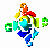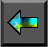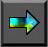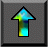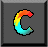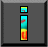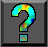Next: 11.4 Mergesort Up: 11 Hypercube Algorithms Previous: 11.2 Vector Reduction

# 11.3 Matrix Transposition

The transposition of a two-dimensional N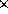N matrix A yields a matrix A' of the same size, in which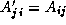. If A and/or A' are distributed between multiple tasks, then execution of the transpose operation may involve communication. We consider here a one-dimensional, columnwise decomposition of the input and output matrices among P tasks. Notice that this transposition requires all-to-all communication.

One commonly used transposition algorithm proceeds in P-1 steps, with each task exchanging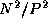data with another task in each step, for a per-processor communication cost of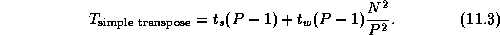This algorithm was used in the convolution example in Section 4.4. An alternative algorithm, described here, uses the hypercube communication template to reduce message startup costs at the expense   of increased data transfer costs. The basic idea is similar to that   used in the recursive halving reduction algorithm, but because the operator used to combine messages in the transpose is ``append'' rather than ``reduce,'' message sizes do not become smaller as the transpose proceeds.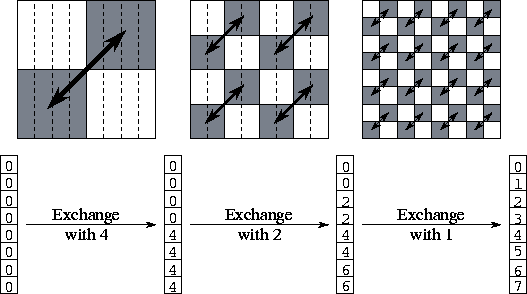Figure 11.3: The three steps of the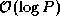matrix transpose algorithm when P=N=8. Initially, each task has a single column of the matrix. After the transpose, each task has a single row. In each step, each task exchanges one half of its data; this data is shaded in the upper part of the figure. The lower part of the figure shows the origin of the eight values held by task 0 at each step of the algorithm. Task 0 sends elements 4--7 in its first message and receives four elements from task 4; these are stored in locations 4--7. In the second step, task 0 exchanges both elements 2--3 (its own) and 6--7 (from task 3) with task 2. In the third step, it exchanges elements 1 (its own), 3 (from task 2), 5 (from task 4), and 7 (from task 6) with task 1.

The algorithm proceeds as follows. Tasks are partitioned into two sets. Corresponding pairs of tasks in the two sets exchange the one half of their data that is destined for tasks in the other set. Tasks 0..(P/2)-1 communicate the lower half of their data, while tasks (P/2)..P-1 communicate the upper half. This partitioning and exchange process is repeated until each set contains a single task. See Figure 11.3 for more details.

As each of the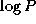messages has size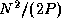, the communication cost is: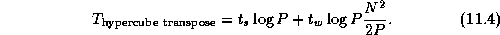A comparison of Equations 11.3 and 11.4 shows that the hypercube algorithm sends about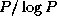fewer messages but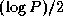times more data. In most situations, the data transfer term dominates, and thealgorithm is to be preferred. However, we can expect thealgorithm to be competitive on small problems and when message startups are expensive and transfer costs are low.Next: 11.4 Mergesort Up: 11 Hypercube Algorithms Previous: 11.2 Vector Reduction